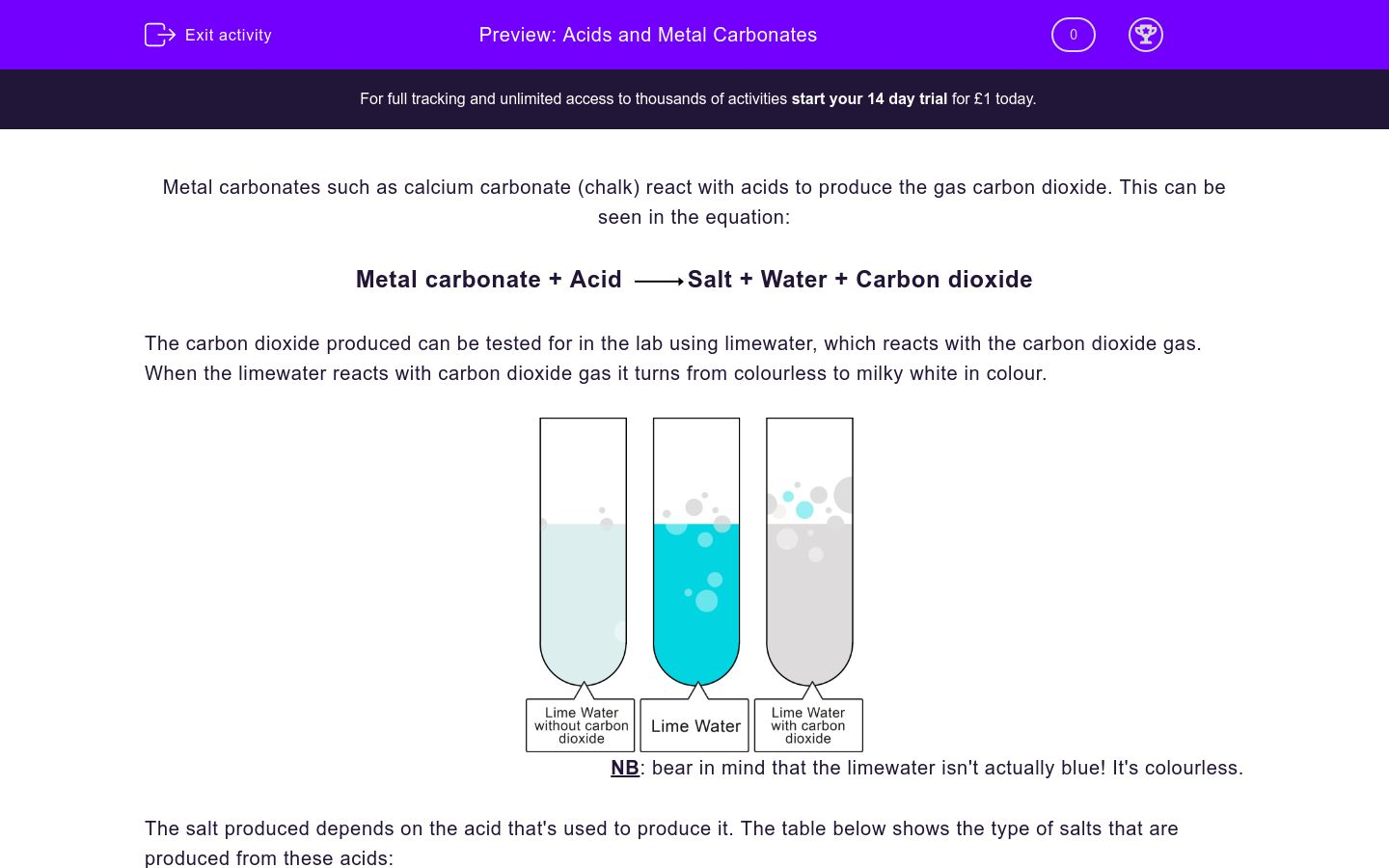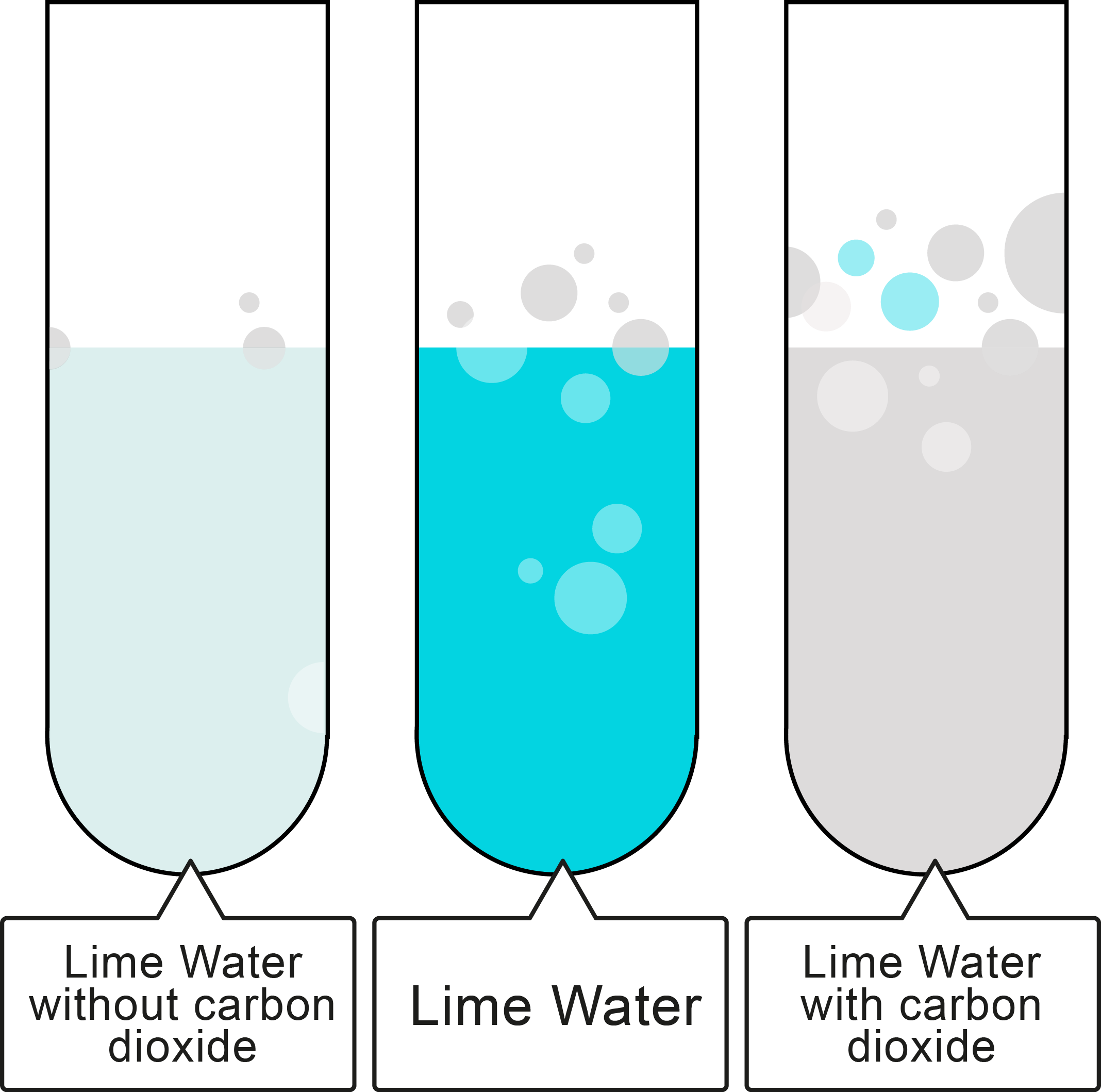# Acids and Metal Carbonates

In this worksheet, students will look at reactions of acids and, specifically, at the reaction of metals carbonates with acids.Key stage:  KS 3

Curriculum topic:   Chemistry: Chemical Reactions

Curriculum subtopic:   Reactions of Acids with Metals

Difficulty level:### QUESTION 1 of 10

Metal carbonates such as calcium carbonate (chalk) react with acids to produce the gas carbon dioxide. This can be seen in the equation:

Metal carbonate + AcidSalt + Water + Carbon dioxide

The carbon dioxide produced can be tested for in the lab using limewater, which reacts with the carbon dioxide gas. When the limewater reacts with carbon dioxide gas it turns from colourless to milky white in colour.NB: bear in mind that the limewater isn't actually blue! It's colourless.

The salt produced depends on the acid that's used to produce it. The table below shows the type of salts that are produced from these acids:

 Acid Name Type of Salt Hydrochloric Acid chloride Sulfuric Acid sulfate Nitric Acid nitrate

The name of the salts produced is better illustrated in an equation:

Hydrochloric Acid

Magnesium carbonate + Hydrochloric acidMagnesium chloride + Water + Carbon dioxide

MgCO3+ 2HClMgCl2 + H2O + CO2

Sulfuric Acid

Calcium carbonate + Sulfuric acidCalcium sulfate + Water + Carbon dioxide

CaCO3+ H2SO4CaSO4 + H2O + CO2

Nitric Acid

Sodium carbonate + Sulfuric acidSodium nitrate + Water + Carbon dioxide

Na2CO3+ 2HNO32NaNO3 + H2O + CO2

What gas is always produced when a metal carbonate reacts with an acid?

hydrogen

carbon dioxide

sulfur dioxide

methane

What is the correct chemical name of chalk?

sodium chloride

carbon dioxide

calcium carbonate

nitric acid

Which of the following compounds would react with an acid to produce carbon dioxide?

 Carbon dioxide produced No carbon dioxide produced Sodium hydroxide Magnesium carbonate Sodium Calcium hydroxide Calcium carbonate

Which of the following reagents can be used to test for carbon dioxide gas?

Benedict's reagent

universal indicator

limewater

iodine

Which of the following is not produced in a reaction between an acid and a metal carbonate?

carbon dioxide

hydrogen

water

salt

Complete the following equation by typing the name of the missing chemical in the text box below.

metal carbonate + ___________salt + water + carbon dioxide

Complete the following equation by selecting the name of the missing chemical from the list below:

Sodium carbonate + _____________sodium sulfate + water + carbon dioxide

hydrochloric acid

sulfuric acid

nitric acid

ethanoic acid

Complete the following equation by selecting the formula of the missing chemical from the list below.

MgCO3 + ___________Mg(NO3)2 + H2O + CO2

H2SO4

HNO3

HCl

CH3COOH

• Question 1

What gas is always produced when a metal carbonate reacts with an acid?

carbon dioxide
EDDIE SAYS
When a metal carbonate reacts with an acid, salt, water and carbon dioxide gas are produced.
• Question 2

What is the correct chemical name of chalk?

calcium carbonate
EDDIE SAYS
Calcium carbonate is the main constituent of chalk. This is definitely worth remembering as chalk, limestone and marble are used so much and all contain calcium carbonate.
• Question 3

Which of the following compounds would react with an acid to produce carbon dioxide?

 Carbon dioxide produced No carbon dioxide produced Sodium hydroxide Magnesium carbonate Sodium Calcium hydroxide Calcium carbonate
EDDIE SAYS
The clue is in the name: carbonates react with acids to produce carbon dioxide gas.
• Question 4

Which of the following reagents can be used to test for carbon dioxide gas?

limewater
EDDIE SAYS
Limewater turns from colourless to milky white in the presence of carbon dioxide gas.
It's worth remembering some other tests too: Universal Indicator tests the pH of a solution, Benedict's reagent tests for reducing sugars and iodine solution can be used to test for starch.
• Question 5

Which of the following is not produced in a reaction between an acid and a metal carbonate?

hydrogen
EDDIE SAYS
Hydrogen is not produced in this reaction, it is produced when a metal reacts with an acid.
• Question 6

Complete the following equation by typing the name of the missing chemical in the text box below.

metal carbonate + ___________salt + water + carbon dioxide

acid
EDDIE SAYS
The reaction of a metal carbonate with an acid produces salt, water and carbon dioxide.
• Question 7

Complete the following equation by selecting the name of the missing chemical from the list below:

Sodium carbonate + _____________sodium sulfate + water + carbon dioxide

sulfuric acid
EDDIE SAYS
The name of the salt gives you the information required to identify the acid used. So, in this case, sodium sulfate is the salt. So that means the acid HAS to be sulfuric acid (call it Hydrogen Sulphate if that helps!).
• Question 8

Complete the following equation by selecting the formula of the missing chemical from the list below.

MgCO3 + ___________Mg(NO3)2 + H2O + CO2

HNO3
EDDIE SAYS
It is important to learn the formulae of hydrochloric acid (HCl), sulfuric acid (H2SO4), and nitric acid (HNO3). So, above, you can see that the salt is magnesium nitrate - the acid must therefore have been nitric acid (call it Hydrogen Nitrate if that helps).
---- OR ----

Sign up for a £1 trial so you can track and measure your child's progress on this activity.

### What is EdPlace?

We're your National Curriculum aligned online education content provider helping each child succeed in English, maths and science from year 1 to GCSE. With an EdPlace account you’ll be able to track and measure progress, helping each child achieve their best. We build confidence and attainment by personalising each child’s learning at a level that suits them.

Get started